# Bihar Board 12th Maths Objective Answers Chapter 11 Three Dimensional Geometry

Bihar Board 12th Maths Objective Questions and Answers

## Bihar Board 12th Maths Objective Answers Chapter 11 Three Dimensional Geometry

Question 1.
Direction cosines of the line that makes equal angles with the three axes in space are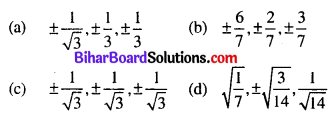(c) $$\pm \frac{1}{\sqrt{3}}, \pm \frac{1}{\sqrt{3}}, \pm \frac{1}{\sqrt{3}}$$

Question 2.
If the direction ratios of a line are 1, -3, 2, then its direction cosines are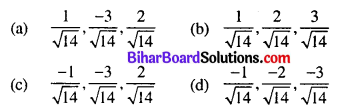(a) $$\frac{1}{\sqrt{14}}, \frac{-3}{\sqrt{14}}, \frac{2}{\sqrt{14}}$$

Question 3.
The cosines of the angle between any two diagonals of a cube is
(a) $$\frac{1}{3}$$
(b) $$\frac{1}{2}$$
(c) $$\frac{2}{3}$$
(d) $$\frac{1}{\sqrt{3}}$$
(a) $$\frac{1}{3}$$

Question 4.
Which of the following is false?
(a) 30°, 45°, 60° can be the direction angles of a line is space.
(b) 90°, 135°, 45° can be the direction angles of a line is space.
(c) 120°, 60°, 45° can be the direction angles of a line in space.
(d) 60°, 45°, 60° can be the direction angles of a line in space.
(a) 30°, 45°, 60° can be the direction angles of a line is space.

Question 5.
A line makes angles α, β and γ with the co-ordinate axes. If α + β = 90°, then γ is equal to
(a) 0°
(b) 90°
(c) 180°
(d) None of these
(b) 90°

Question 6.
If a line makes an angle θ1, θ2, θ3 with the axis respectively, then cos 2θ1 + cos 2θ2 + cos 2θ3 =
(a) -4
(b) -2
(c) -3
(d) -1
(d) -1

Question 7.
The coordinates of a point P are (3, 12, 4) w.r.t. origin O, then the direction cosines of OP are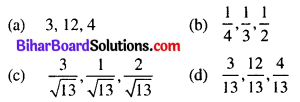(d) $$\frac{3}{13}, \frac{12}{13}, \frac{4}{13}$$

Question 8.
Find the direction cosines of the line joining A(0, 7, 10) and B(-1, 6, 6).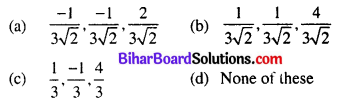(b) $$\frac{1}{3 \sqrt{2}}, \frac{1}{3 \sqrt{2}}, \frac{4}{3 \sqrt{2}}$$

Question 9.
The direction cosines of a line passing through two points P(x1, y1, z1) and Q(x2, y2, z2) are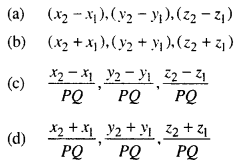(c) $$\frac{x_{2}-x_{1}}{P Q}, \frac{y_{2}-y_{1}}{P Q}, \frac{z_{2}-z_{1}}{P Q}$$

Question 10.
The equation of a line which passes through the point (1, 2, 3) and is parallel to the vector $$3 \hat{i}+2 \hat{j}-2 \hat{k}$$, is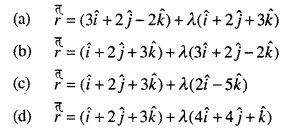(b) $$r=(\hat{i}+2 \hat{j}+3 \hat{k})+\lambda(3 \hat{i}+2 \hat{j}-2 \hat{k})$$

Question 11.
The equation of line passing through the point (-3, 2, -4) and equally inclined to the axes are
(a) x – 3 = y + 2 = z – 4
(b) x + 3 = y – 2 = z + 4
(c) $$\frac{x+3}{1}=\frac{y-2}{2}=\frac{z+4}{3}$$
(d) None of these
(b) x + 3 = y – 2 = z + 4

Question 12.
If l, m and n are the direction cosines of line l, then the equation of the line (l) passing through (x1, y1, z1) is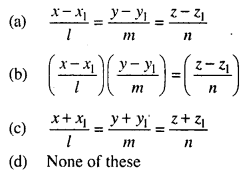(a) $$\frac{x-x_{1}}{l}=\frac{y-y_{1}}{m}=\frac{z-z_{1}}{n}$$

Question 13.
In the figure, a be the position vector of the point A with respect to the origin O. l is a line parallel to a
vector b. The vector equation of line l is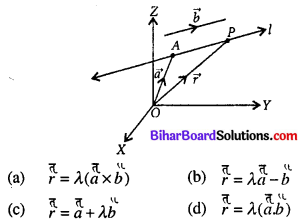(c) r = a + λb

Question 14.
The certesian equation of the line l when it passes through the point (x1, y1, z1) and parallel to the vector
b = $$a \hat{i}+b \hat{j}+c \hat{k}$$, is
(a) x – x1 = y – y1 = z – z1
(b) x + x1 = y + y1 = z + z1
(c) $$\frac{x+x_{1}}{a}=\frac{y+y_{1}}{b}=\frac{z+z_{1}}{c}$$
(d) $$\frac{x-x_{1}}{a}=\frac{y-y_{1}}{b}=\frac{z-z_{1}}{c}$$
(d) $$\frac{x-x_{1}}{a}=\frac{y-y_{1}}{b}=\frac{z-z_{1}}{c}$$

Question 15.
The equation of the straight line passing through the point (a, b, c) and parallel to Z-axis is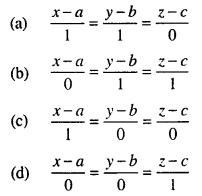(d) $$\frac{x-a}{0}=\frac{y-b}{0}=\frac{z-c}{1}$$

Question 16.
The coordinates of a point on the line $$\frac{x+2}{3}=\frac{y+1}{2}=\frac{z-3}{2}$$ at a distance of $$\frac{6}{\sqrt{12}}$$ from the point (1, 2, 3) is
(a) (56, 43, 111)
(b) $$\left(\frac{56}{17}, \frac{43}{17}, \frac{111}{17}\right)$$
(c) (2, 1, 3)
(d) (-2, -1, -3)
(b) $$\left(\frac{56}{17}, \frac{43}{17}, \frac{111}{17}\right)$$

Question 17.
Find the coordinatets of the point where the line through the points (5, 1, 6) and (3, 4, 1) crosses the yz-plane.
(a) $$\left(0,-\frac{17}{2}, \frac{13}{2}\right)$$
(b) $$\left(0, \frac{17}{2},-\frac{13}{2}\right)$$
(c) $$\left(10, \frac{19}{2}, \frac{13}{2}\right)$$
(d) (0, 17, 13)
(b) $$\left(0, \frac{17}{2},-\frac{13}{2}\right)$$

Question 18.
The point A(1, 2, 3), B(-1, -2, -1) and C(2, 3, 2) are three vertices of a parallelogram ABCD. Find the equation of CD.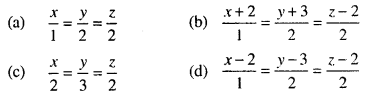(d) $$\frac{x-2}{1}=\frac{y-3}{2}=\frac{z-2}{2}$$

Question 19.
The equation of the line joining the points (-3, 4, 11) and (1, -2, 7) is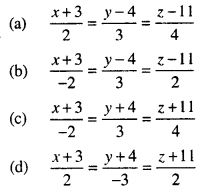(b) $$\frac{x+3}{-2}=\frac{y-4}{3}=\frac{z-11}{2}$$

Question 20.
The vector equation of the line through the points A(3, 4, -7) and B(1, -1, 6) is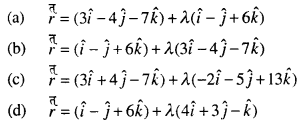(c) $$r=(3 \hat{i}+4 \hat{j}-7 \hat{k})+\lambda(-2 \hat{i}-5 \hat{j}+13 \hat{k})$$

Question 21.
The vactor equation of the symmetrical form of equation of straight line $$\frac{x+5}{3}=\frac{y+4}{7}=\frac{z-6}{2}$$ is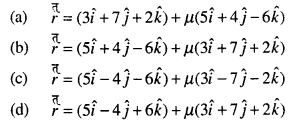(d) $$r=(5 \hat{i}-4 \hat{j}+6 \hat{k})+\mu(3 \hat{i}+7 \hat{j}+2 \hat{k})$$

Question 22.
Vector equation of the line 6x – 3 = 3y + 4 = 2z – 2 is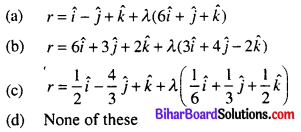(c) $$r=\frac{1}{2} \hat{i}-\frac{4}{3} \hat{j}+\hat{k}+\lambda\left(\frac{1}{6} \hat{i}+\frac{1}{3} \hat{j}+\frac{1}{2} \hat{k}\right)$$

Question 23.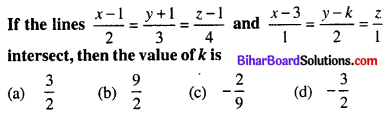(b) $$\frac{9}{2}$$

Question 24.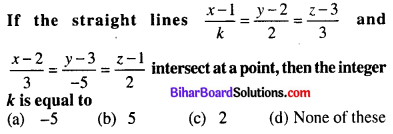(a) -5

Question 25.
The angle between the straight lines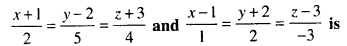(a) 45°
(b) 30°
(c) 60°
(d) 90°
(d) 90°

Question 26.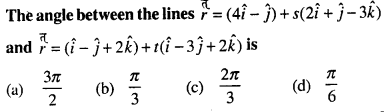(d) $$\frac{\pi}{6}$$

Question 27.
The angle between the line 2x = 3y = -z and 6x = -y = -4z is
(a) 30°
(b) 45°
(c) 90°
(d) 0°
(c) 90°

Question 28.
The angle between the lines 3x = 6y = 2z and $$\frac{x-2}{-5}=\frac{y-1}{7}=\frac{z-3}{1}$$ is
(a) $$\frac{\pi}{6}$$
(b) $$\frac{\pi}{4}$$
(c) $$\frac{\pi}{3}$$
(d) $$\frac{\pi}{2}$$
(d) $$\frac{\pi}{2}$$

Question 29.
Find the angle between the pair of lines given by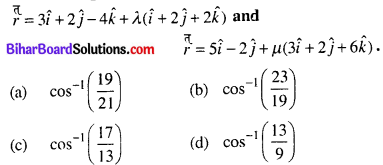(a) $$\cos ^{-1}\left(\frac{19}{21}\right)$$

Question 30.
The angle between the lines x = 1, y = 2 and y = -1, z = 0 is
(a) 90°
(b) 30°
(c) 60°
(d) 0°
(a) 90°

Question 31.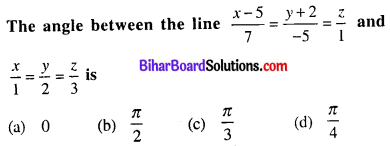(b) $$\frac{\pi}{2}$$

Question 32.
The angle between the lines passing through the points (4, 7, 8), (2, 3, 4) and (-1, -2, 1), (1, 2, 5) is
(a) 0
(b) $$\frac{\pi}{2}$$
(c) $$\frac{\pi}{4}$$
(d) $$\frac{\pi}{6}$$
(a) 0

Question 33.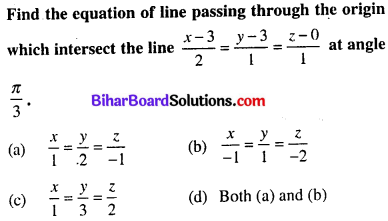(d) Both (a) and (b)

Question 34.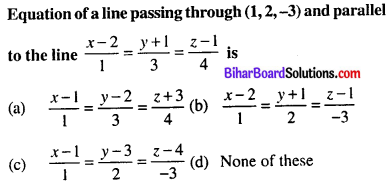(a) $$\frac{x-1}{1}=\frac{y-2}{3}=\frac{z+3}{4}$$

Question 35.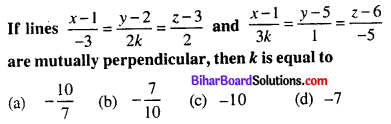(a) $$-\frac{10}{7}$$

Question 36.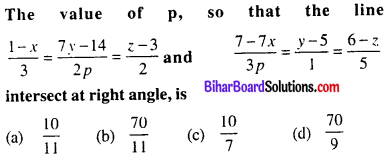(b) $$\frac{70}{11}$$

Question 37.
The shortest distance between the lines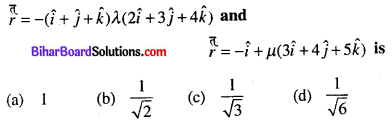(d) $$\frac{1}{\sqrt{6}}$$

Question 38.
The shortest distance between the lines $$\frac{x-3}{3}=\frac{y-8}{-1}=\frac{z-3}{1}$$ and $$\frac{x+3}{-3}=\frac{y+7}{2}=\frac{z-6}{4}$$ is equal
(a) 3√30
(b) √30
(c) 2√30
(d) None of these
(a) 3√30

Question 39.
The shortest distance between the lines x = y = z and x + 1 – y = $$\frac{z}{0}$$ is
(a) $$\frac{1}{2}$$
(b) $$\frac{1}{\sqrt{2}}$$
(c) $$\frac{1}{\sqrt{3}}$$
(d) $$\frac{1}{\sqrt{6}}$$
(d) $$\frac{1}{\sqrt{6}}$$

Question 40.
The shortest distance between the lines x = y + 2 = 6z – 6 and x + 1 = 2y = -12z is
(a) $$\frac{1}{2}$$
(b) 2
(c) 1
(d) $$\frac{3}{2}$$
(b) 2

Question 41.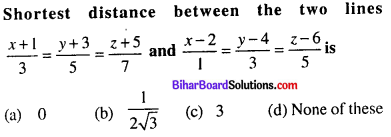(a) 0

Question 42.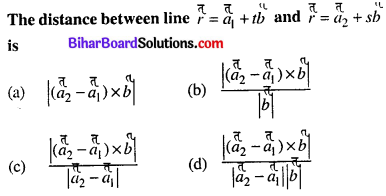(b) $$\frac{\left|\left(\tilde{a}_{2}-a_{1}\right) \times b\right|}{|b|}$$

Question 43.(b) $$\sqrt{\frac{59}{7}}$$

Question 44.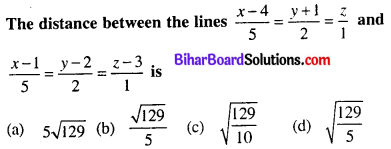(d) $$\sqrt{\frac{129}{5}}$$

Question 45.
The direction cosines of the unit vector perpendicular to the plane $$r \cdot(6 \hat{i}-3 \hat{j}-2 \hat{k})+1=0$$ passing through the origin are
(a) $$\frac{6}{7}, \frac{3}{7}, \frac{2}{7}$$
(b) 6, 3, 2
(c) $$-\frac{6}{7}, \frac{3}{7}, \frac{2}{7}$$
(d) -6, 3, 2
(c) $$-\frac{6}{7}, \frac{3}{7}, \frac{2}{7}$$

Question 46.
The coordinate of the foot of perpendicular drawn from origin to the plane 2x – 3y + 4z – 6 = 0 is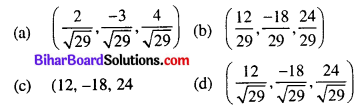(d) $$\left(\frac{12}{\sqrt{29}}, \frac{-18}{\sqrt{29}}, \frac{24}{\sqrt{29}}\right)$$

Question 47.
The vector equation of a plane which is at a distance of 7 units from the origin and normal to the vector
$$3 \hat{i}+5 \hat{j}-6 \hat{k}$$ is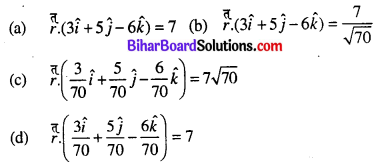(d) $$r \cdot\left(\frac{3 \hat{i}}{70}+\frac{5 \hat{j}}{70}-\frac{6 \hat{k}}{70}\right)=7$$

Question 48.
Find the vector equation of the plane which is at a distance of 8 units from the origin and which is normal to the vector $$2 \hat{i}+\hat{j}+2 \hat{k}$$.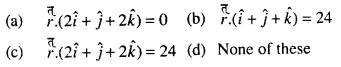(c) $$r_{\cdot}(2 \hat{i}+\hat{j}+2 \hat{k})=24$$

Question 49.
Find the length of perpendicular from the origin to the plane $$r(3 \hat{i}-4 \hat{j}+12 \hat{k})$$.
(a) $$\frac{5}{13}$$
(b) $$\frac{5}{\sqrt{13}}$$
(c) $$\frac{5}{23}$$
(d) $$\frac{\sqrt{5}}{13}$$
(a) $$\frac{5}{13}$$

Question 50.
The equation of the plane passing through three non- collinear points with position vectors a, b, c is
(a) r.(b × c + c × a + a × b) = 0
(b) r.(b × c + c × a + a × b) = [abc]
(c) r.(a × (b + c)) = [abc]
(d) r.(a + b + c) = 0
(b) r.(b × c + c × a + a × b) = [abc]

Question 51.
Equation of the plane passing through three points A, B, C with position vectors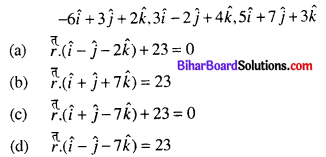(a) $$\pi(\hat{i}-\hat{j}-2 \hat{k})+23=0$$

Question 52.
Four points (0, -1, -1) (-4, 4, 4) (4, 5, 1) and (3, 9, 4) are coplanar. Find the equation of the plane containing them.
(a) 5x + 7y + 11z – 4 =0
(b) 5x – 7y + 11z + 4 = 0
(c) 5x – 7y – 11z – 4 = 0
(d) 5x + 7y – 11z + 4 = 0
(b) 5x – 7y + 11z + 4 = 0

Question 53.
Find the equation of plane passing through the points P(1, 1, 1), Q(3, -1, 2), R(-3, 5, -4).
(a) x + 2y = 0
(b) x – y = 2
(c) -x + 2y = 2
(d) x + y = 2
(d) x + y = 2

Question 54.
The vector equation of the plane passing through the origin and the line of intersection of the plane r.a = λ and r.b = µ is
(a) r.(λa – µb) = 0
(b) r.(λb – µa) = 0
(c) r.(λa + µb)= 0
(d) r.(λb + µa) = 0
(b) r.(λb – µa) = 0

Question 55.
The vector equation of a plane passing through the intersection of the planes $$r_{\cdot}(\hat{i}+\hat{j}+\hat{k})=6$$ and $$r_{\cdot}(2 \hat{i}+3 \hat{j}+4 \hat{k})=-5$$ and the point (1, 1, 1) is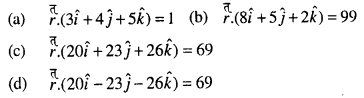(c) $$r_{\cdot}(20 \hat{i}+23 \hat{j}+26 \hat{k})=69$$

Question 56.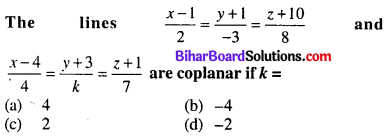(b) -4

Question 57.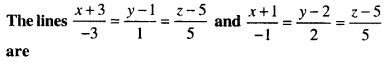(a) coplanar
(b) non-coplanar
(c) perpendicular
(d) None of the above
(a) coplanar

Question 58.
The angle between the planes 3x + 2y + z – 5 = 0 and x + y – 2z – 3 = 0 is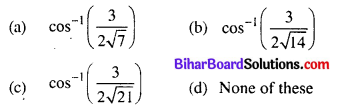(c) $$\cos ^{-1}\left(\frac{3}{2 \sqrt{21}}\right)$$

Question 59.
The equation of the plane through the point (0, -4, -6) and (-2, 9, 3) and perpendicular to the plane x – 4y – 2z = 8 is
(a) 3x + 3y – 2z = 0
(b) x – 2y + z = 2
(c) 2x + y – z = 2
(d) 5x – 3y + 2z = 0
(c) 2x + y – z = 2

Question 60.
The angle between the planes $$r \cdot(\hat{i}+2 \hat{j}+\hat{k})=4$$ and $$r(-\hat{i}+\hat{j}+2 \hat{k})=9$$ is
(a) 30°
(b) 60°
(c) 45°
(d) None of these
(b) 60°

Question 61.
The angle between the panes x + y = 0 and y – z = 1 is
(a) $$\frac{\pi}{6}$$
(b) $$\frac{\pi}{4}$$
(c) $$\frac{\pi}{3}$$
(d) $$\frac{\pi}{2}$$
(c) $$\frac{\pi}{3}$$

Question 62.
If the angle between the planes 2x – y + 2z = 3 and 3x + 6y + cz = 4 is $$\cos ^{-1}\left(\frac{4}{21}\right)$$, then c2 =
(a) 1
(b) 4
(c) 9
(d) 5
(b) 4

Question 63.
The distance of the plane 2x – 3y + 4z – 6 = 0 from the origin is A. Here, A refers to
(a) 6
(b) -6
(c) $$-\frac{6}{\sqrt{29}}$$
(d) $$\frac{6}{\sqrt{29}}$$
(b) -6

Question 64.
Find the length of perpendicular from origin to the plane $$r \cdot(3 \hat{i}-4 \hat{j}-12 \hat{k})+39=0$$
(a) 1
(b) 3
(c) $$\frac{1}{7}$$
(d) None of these
(b) 3

Question 65.
The distance of the origin from the plane through the points (1, 1, 0), (1, 2, 1) and (-2, 2, -1) is
(a) $$\frac{3}{\sqrt{11}}$$
(b) $$\frac{5}{\sqrt{22}}$$
(c) 3
(d) $$\frac{4}{\sqrt{22}}$$
(b) $$\frac{5}{\sqrt{22}}$$

Question 66.
The angle θ between the line r = a + λb is given by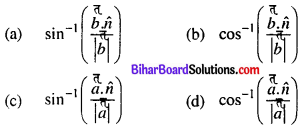(a) $$\sin ^{-1}\left(\frac{\tilde{h}_{\hat{\pi}}^{\pi}}{|\vec{b}|}\right)$$

Question 67.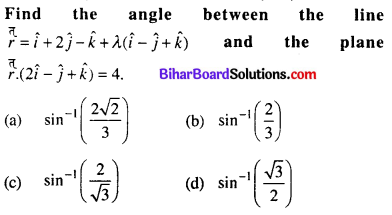(a) $$\sin ^{-1}\left(\frac{2 \sqrt{2}}{3}\right)$$

Question 68.
The angle between the straight line $$\frac{x-1}{2}=\frac{y+3}{-1}=\frac{z-5}{2}$$ and the plane 4x – 2y + 4z = 9 is
(a) 60°
(b) 90°
(c) 45°
(d) 30°
(b) 90°

Question 69.
Distance of the point (α, β, γ) from y-axis is
(a) β
(b) |β|
(c) |β| + |γ|
(d) $$\sqrt{\alpha^{2}+\gamma^{2}}$$
(d) $$\sqrt{\alpha^{2}+\gamma^{2}}$$

Question 70.
The distance of the plane $$r \cdot\left(\frac{2}{7} \hat{i}+\frac{3}{7} \hat{j}-\frac{6}{7} \hat{k}\right)=1$$ from the origin is
(a) 1
(b) 7
(c) $$\frac{1}{7}$$
(d) None of these
(a) 1

Question 71.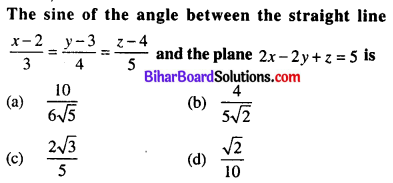(d) $$\frac{\sqrt{2}}{10}$$

Question 72.
The reflection of the point (α, β, γ) in the xy-plane is
(a) (α, β, 0)
(b) (0, 0, γ)
(c) (-α, -β, -γ)
(d) (α, β, -y)
(d) (α, β, -y)

Question 73.
The area of the quadrilateral ABCD, where A(0, 4, 1), B(2, 3, -1), C(4, 5, 0) and D(2, 6, 2), is equal to
(a) 9 sq. units
(b) 18 sq. units
(c) 27 sq. units
(d) 81 sq. units